Aniruddha Bhandari — November 26, 2020

## Overview

• Apache spark is amongst the favorite tools for any big data engineer
• Learn Spark Optimization with these 8 tips
• By no means is this list exhaustive. Feel free to add any spark optimization technique that we missed in the comments below

## Introduction

The biggest hurdle encountered when working with Big Data isn’t of accomplishing a task, but of accomplishing it in the least possible time with the fewest of resources. That’s where Apache Spark comes in with amazing flexibility to optimize your code so that you get the most bang for your buck!In this article, we will discuss 8 Spark optimization tips that every data engineering beginner should be aware of. Most of these are simple techniques that you need to swap with the inefficient code that you might be using unknowingly. While others are small tweaks that you need to make to your present code to be a Spark superstar. So let’s get started without further ado!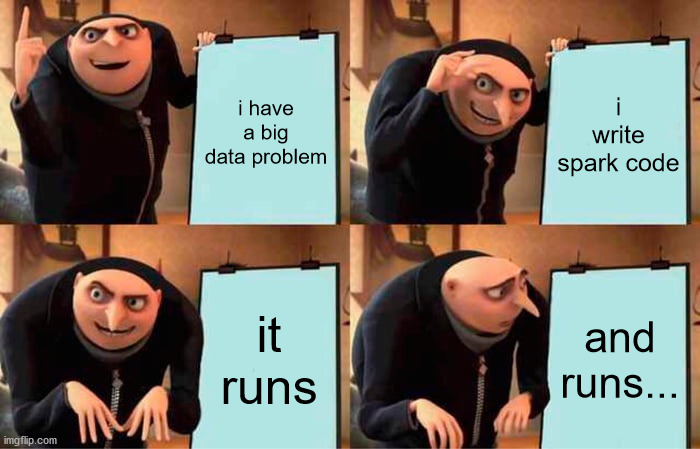If you are a total beginner and have got no clue what Spark is and what are its basic components, I suggest going over the following articles first:

1. Don’t Collect Data
2. Persistence is the Key
3. Avoid Groupbykey
4. Aggregate with Accumulators
6. Be Shrewd with Partitioning
8. Don’t Repartition your data – Coalesce it

## 1. Don’t Collect Data

As a data engineer beginner, we start out with small data, get used to a few commands, and stick to them, even when we move on to working with Big Data. One such command is the collect() action in Spark.

When we call the collect action, the result is returned to the driver node. This might seem innocuous at first. But if you are working with huge amounts of data, then the driver node might easily run out of memory.

```# Load data
# Collect
df.collect()```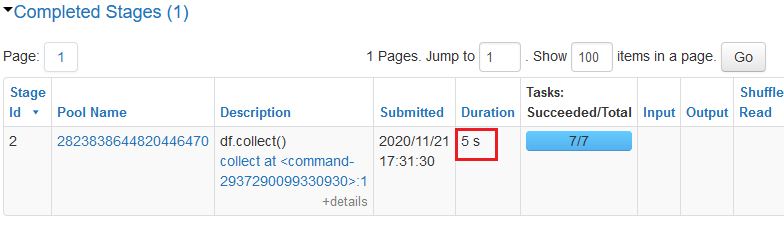So, what should we use instead?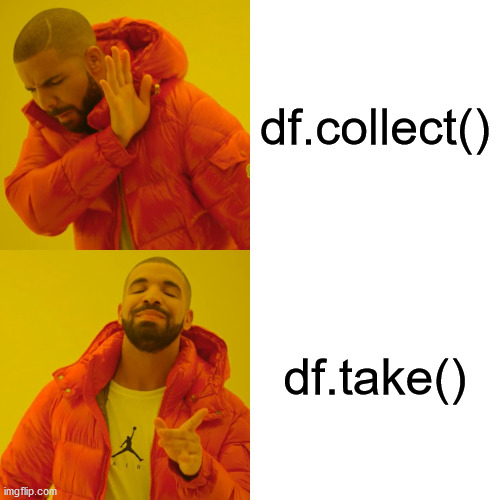One great way to escape is by using the take() action. It scans the first partition it finds and returns the result. As simple as that! For example, if you just want to get a feel of the data, then take(1) row of data.

`df.take(1)`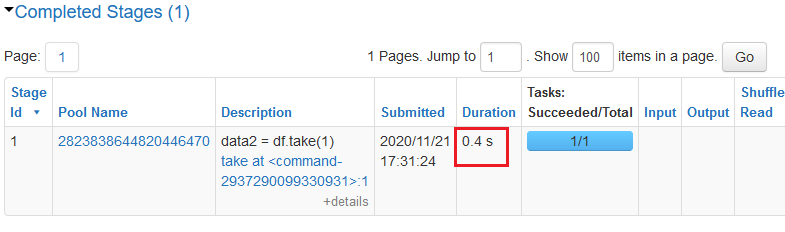This is much more efficient than using collect!

## 2. Persistence is the Key

When you start with Spark, one of the first things you learn is that Spark is a lazy evaluator and that is a good thing. But it could also be the start of the downfall if you don’t navigate the waters well. What do I mean?

Well, suppose you have written a few transformations to be performed on an RDD. Now each time you call an action on the RDD, Spark recomputes the RDD and all its dependencies. This can turn out to be quite expensive.

```l1 = [1, 2, 3, 4]

rdd1 = sc.parallelize(l1)
rdd2 = rdd1.map(lambda x: x*x)
rdd3 = rdd2.map(lambda x: x+2)

print(rdd3.count())
print(rdd3.collect())```

When I call count(), all the transformations are performed and it takes 0.1 s to complete the task.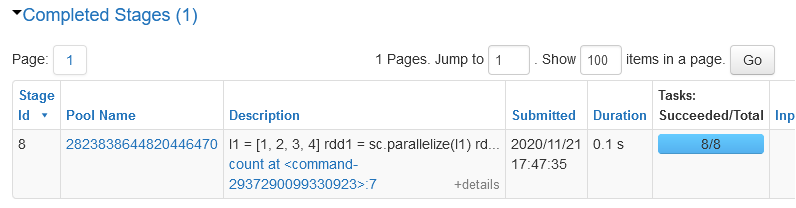When I call collect(), again all the transformations are called and it still takes me 0.1 s to complete the task.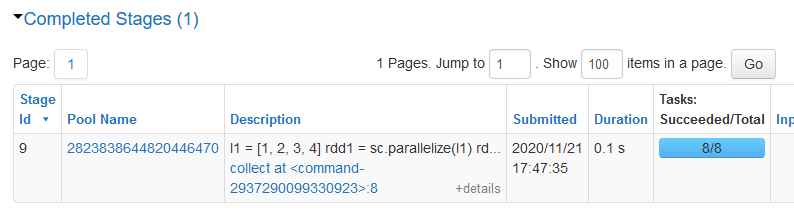So how do we get out of this vicious cycle? Persist!```from pyspark import StorageLevel

# By default cached to memory and disk
rdd3.persist(StorageLevel.MEMORY_AND_DISK)

# before rdd is persisted
print(rdd3.count())
# after rdd is persisted
print(rdd3.collect())```

In our previous code, all we have to do is persist in the final RDD. This way when we first call an action on the RDD, the final data generated will be stored in the cluster. Now, any subsequent use of action on the same RDD would be much faster as we had already stored the previous result.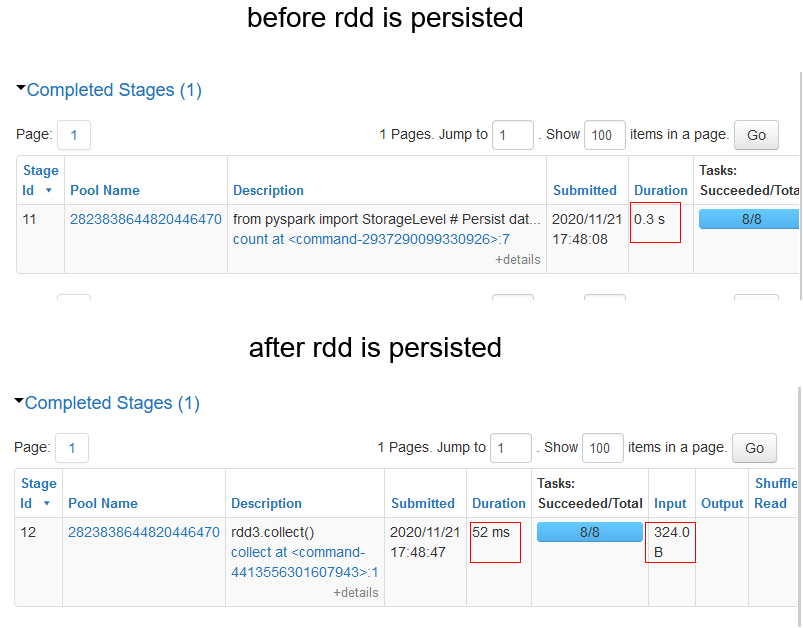Note – Here, we had persisted the data in memory and disk. But there are other options as well to persist the data.

## 3. Avoid Groupbykey

When you started your data engineering journey, you would have certainly come across the word counts example.

```# List of sample sentences
text_list = ["this is a sample sentence", "this is another sample sentence", "sample for a sample test"]

# Create an RDD
rdd = sc.parallelize(text_list)

# Split sentences into words using flatMap
rdd_word = rdd.flatMap(lambda x: x.split(" "))

# Create a paired-rdd
rdd_pair = rdd_word.map(lambda x: (x, 1))

# Count occurence per word using groupbykey()
rdd_group = rdd_pair.groupByKey()
rdd_group_count = rdd_group.map(lambda x:(x, len(x)))
rdd_group_count.collect()```

But why bring it here? Well, it is the best way to highlight the inefficiency of groupbykey() transformation when working with pair-rdds.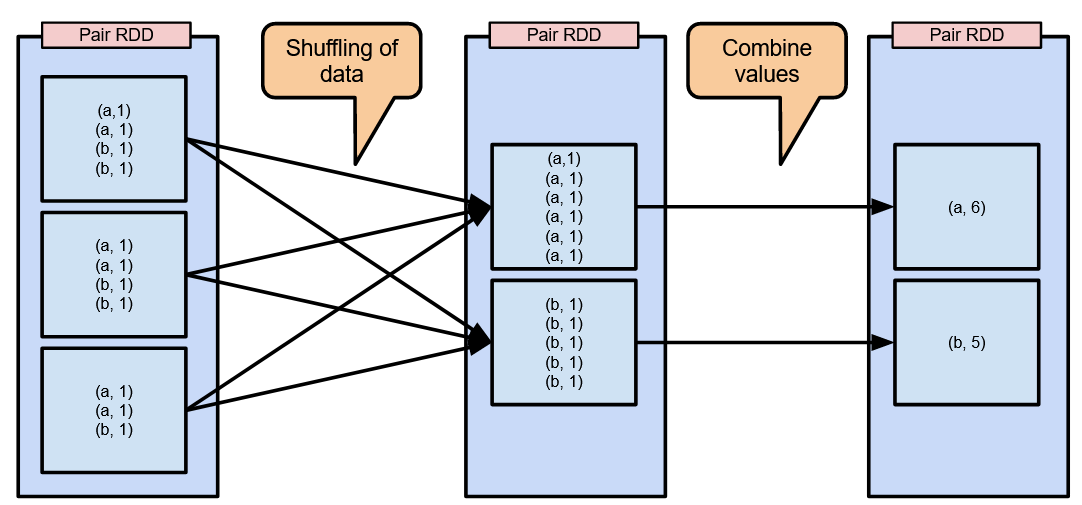Groupbykey shuffles the key-value pairs across the network and then combines them. With much larger data, the shuffling is going to be much more exaggerated. So, how do we deal with this? Reducebykey!

Reducebykey on the other hand first combines the keys within the same partition and only then does it shuffle the data.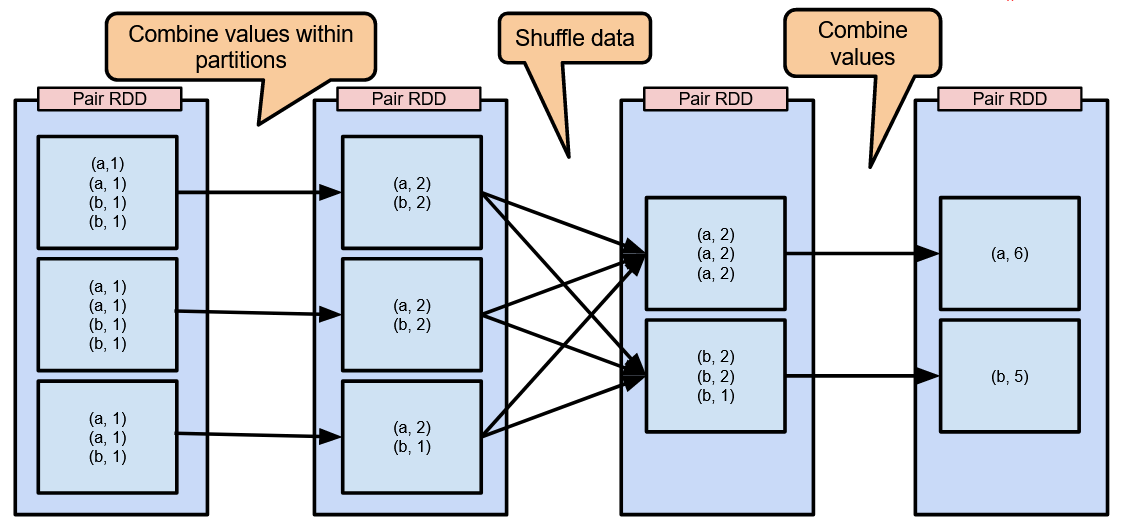Here is how to count the words using reducebykey()

```# Count occurence per word using reducebykey()
rdd_reduce = rdd_pair.reduceByKey(lambda x,y: x+y)
rdd_reduce.collect()```

This leads to much lower amounts of data being shuffled across the network.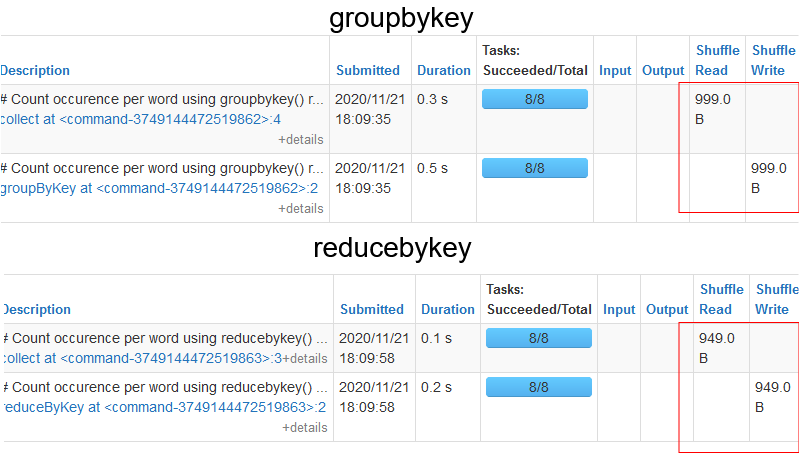As you can see, the amount of data being shuffled in the case of reducebykey is much lower than in the case of groupbykey.## 4. Aggregate with Accumulators

Suppose you want to aggregate some value. This can be done with simple programming using a variable for a counter.

```file = sc.textFile("/FileStore/tables/log.txt")

# variable counter
warningCount = 0

def extractWarning(line):
global warningCount
if ("WARNING" in line):
warningCount +=1

lines = file.flatMap(lambda x: x.split(","))
lines.foreach(extractWarning)

# output variable
warningCount```

But there is a caveat here.

When we try to view the result on the driver node, then we get a 0 value. This is because when the code is implemented on the worker nodes, the variable becomes local to the node. This means that the updated value is not sent back to the driver node. To overcome this problem, we use accumulators.Accumulators have shared variables provided by Spark. They are used for associative and commutative tasks. For example, if you want to count the number of blank lines in a text file or determine the amount of corrupted data then accumulators can turn out to be very helpful.

```file = sc.textFile("/FileStore/tables/log.txt")

# accumulator
warningCount = sc.accumulator(0)

def extractWarning(line):
global warningCount
if ("WARNING" in line):
warningCount +=1

lines = file.flatMap(lambda x: x.split(","))
lines.foreach(extractWarning)

# accumulator value
warningCount.value  # output 4```

One thing to be remembered when working with accumulators is that worker nodes can only write to accumulators. But only the driver node can read the value.

Just like accumulators, Spark has another shared variable called the Broadcast variable. They are only used for reading purposes that get cached in all the worker nodes in the cluster. This comes in handy when you have to send a large look-up table to all nodes.

Assume a file containing data containing the shorthand code for countries (like IND for India) with other kinds of information. You have to transform these codes to the country name. This is where Broadcast variables come in handy using which we can cache the lookup tables in the worker nodes.

```# lookup
country = {"IND":"India","USA":"United States of America","SA":"South Africa"}

# data
userData = [("Johnny","USA"),("Faf","SA"),("Sachin","IND")]

# create rdd
rdd_data = sc.parallelize(userData)

def convert(code):

# transformation
output = rdd_data.map(lambda x: (x, convert(x)))

# action
output.collect()```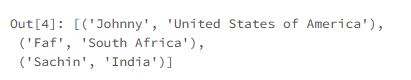## 6. Be shrewd with Partitioning

One of the cornerstones of Spark is its ability to process data in a parallel fashion. Spark splits data into several partitions, each containing some subset of the complete data. For example, if a dataframe contains 10,000 rows and there are 10 partitions, then each partition will have 1000 rows.

The number of partitions in the cluster depends on the number of cores in the cluster and is controlled by the driver node. When Spark runs a task, it is run on a single partition in the cluster. You can check out the number of partitions created for the dataframe as follows:

`df.rdd.getNumPartitions()`

However, this number is adjustable and should be adjusted for better optimization.

Choose too few partitions, you have a number of resources sitting idle.

Choose too many partitions, you have a large number of small partitions shuffling data frequently, which can become highly inefficient.

So what’s the right number?According to Spark, 128 MB is the maximum number of bytes you should pack into a single partition. So, if we have 128000 MB of data, we should have 1000 partitions. But this number is not rigid as we will see in the next tip.

The number of partitions throughout the Spark application will need to be altered. If you started with 100 partitions, you might have to bring them down to 50. But why would we have to do that?

For example, you read a dataframe and create 100 partitions. Next, you filter the data frame to store only certain rows. Now, the amount of data stored in the partitions has been reduced to some extent. Therefore, it is prudent to reduce the number of partitions so that the resources are being used adequately.

But how to adjust the number of partitions?

The repartition() transformation can be used to increase or decrease the number of partitions in the cluster.

```import numpy as np

# data
l1 = np.arange(13)

# rdd
rdd = sc.parallelize(l1)

# check data per partition
print(rdd.glom().collect())

# increase partitions
print(rdd.repartition(10).glom().collect())```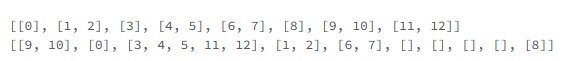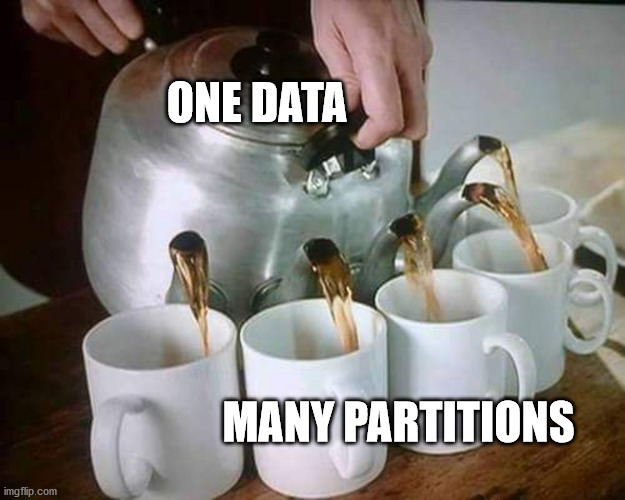When repartition() adjusts the data into the defined number of partitions, it has to shuffle the complete data around in the network. Although this excessive shuffling is unavoidable when increasing the partitions, there is a better way when you are reducing the number of partitions.

## 8. Don’t Repartition your data – Coalesce it

In the last tip, we discussed that reducing the number of partitions with repartition is not the best way to do it. Why?Repartition shuffles the data to calculate the number of partitions. However, we don’t want to do that. To avoid that we use coalesce(). It reduces the number of partitions that need to be performed when reducing the number of partitions.

```print(rdd.glom().collect())
# repartition
print(rdd.repartition(4).glom().collect())
# coalesce
print(rdd.coalesce(4).glom().collect())```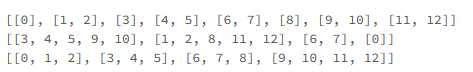The repartition algorithm does a full data shuffle and equally distributes the data among the partitions. It does not attempt to minimize data movement like the coalesce algorithm.

Note: Coalesce can only decrease the number of partitions.

## Endnotes

Hopefully, by now you realized why some of your Spark tasks take so long to execute and how optimization of these spark tasks work.

By no means should you consider this an ultimate guide to Spark optimization, but merely as a stepping stone because there are plenty of others that weren’t covered here. We will probably cover some of them in a separate article.

But till then, do let us know your favorite Spark optimization tip in the comments below, and keep optimizing!Articles to further your knowledge of Spark: